Visualization of the Expansion Theorem

for the Krall Polynomials

in a Weighted Sobolev Space

Rolf Vonhoff

March 11, 2000

1. Synopsis:

The study of orthogonal polynomials within the framework of the spectral theory of differential operators brings together two branches of mathematics in such a manner that advantages for development are to be discerned in both areas ( [EL], p. 21 ) . On the one hand, the classical differential operators with orthogonal polynomial solutions present excellent examples for the spectral theory of singular differential operators in Hilbert space, in which the eigenfunctions are explicitly known. On the other hand, we understand many properties of orthogonal polynomials better, if we observe that these polynomials are the eigenfunctions of an unbounded self-adjoint Hilbert space operator.

In the paper [V2] and in the present work we study the Krall polynomials which have been discovered in 1981 by Lance L. Littlejohn ( [L1]). These polynomials are orthogonal both in a space L^2([-1,1],m) of Lebesgue-Stieltjes-integrable functions and in a weighted Sobolev space W(-1,1) of the order three. On the interval (-1,1) the Krall polynomials are eigenfunctions of the sixth-order Legendre type differential equation, which gives the connection to the spectral theory of singular differential operators. In [V2] we have considered this differential operator in the weighted Sobolev space W(-1,1). Especially we have found a self-adjoint realization of the corresponding maximal operator by means of two boundary conditions. As a main result we prove theorems on eigenfunction expansions both with respect to the weighted Sobolev norm and with respect to locally uniform convergence.

In this work we put the main focus on visualizing some of the results in [V2]. The calculations and plots here are made with the program Maple V Release 5, actually, this whole document is a Maple worksheet. At first, we take a look at the Krall polynomials and display their graphs. After having introduced the weighted Sobolev scalar product in the space W(-1,1) we define the Fourier series corresponding to the Krall polynomials. We then visualize the property of the Krall polynomials that their Fourier series approximates the functions in W(-1,1) locally uniformly and simultaneously up to the second derivative. Some general remarks on the interplay of orthogonal polynomials and spectral theory with a few historical notes conclude our work.

2. References:

[EL] W.N. Everitt and L.L. Littlejohn: Orthogonal Polynomials and Spectral Theory: A Survey , Orthogonal Polynomials and their Applications, C. Brezinski, L. Gori and A. Ronveaux (Editors), Proceedings of the third International Symposium held in Erice, Italy 1990 (1991), pp. 21-55.

The Krall polynomials have been discovered by Lance L. Littlejohn in 1981 and they are named after H.L. Krall (see [L1] and [L2]). Certain remarks indicate that these polynomials already had been discovered by H.L. Krall himself and that they were rediscovered by Littlejohn (cf. the note on p. 115 of the paper [LK] by Littlejohn and A.M. Krall), but no publication of H.L. Krall on these polynomials is known.

Definition of the Krall polynomials :
(n - degree of the polynomial, A,B - positive parameters, x - independent variable)

This definition gives the general Krall polynomial K(n,A,B, . ) of degree n corresponding to the parameters A,B>0. For concrete evaluations, for example when plotting the polynomials, we choose special values for A,B, e.g. A=1, B=10.

> K := ((n,A,B,x) -> sum('(-1)^floor(j/2)*(2*n-j)!/(2^(n+1)*(n-floor((j+1)/2))!*floor(j/2)!*(n-j)!*(n*(n+1)+A+B))* ((1+(-1)^j)/2 * (n^2*(n^2+2*A+2*B-1)+4*A*B+2*j*(n*(n+1)+A+B)) + (1-(-1)^j)/2 * 4*(B-A)) *x^(n-j)','j'=0..n));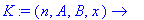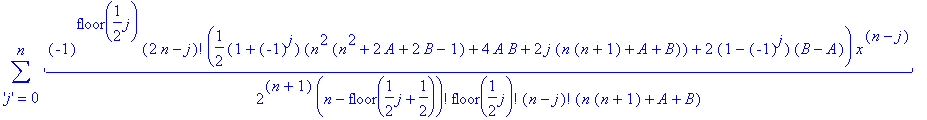In order to give an idea of the construction of these polynomials, we shall list the first four Krall polynomials explicitly.

> array(1..4,1..1,[[K(0,A,B,x)],[sort(simplify(K(1,A,B,x)))],
[sort(simplify(K(2,A,B,x)))],[sort(simplify(K(3,A,B,x)))]]);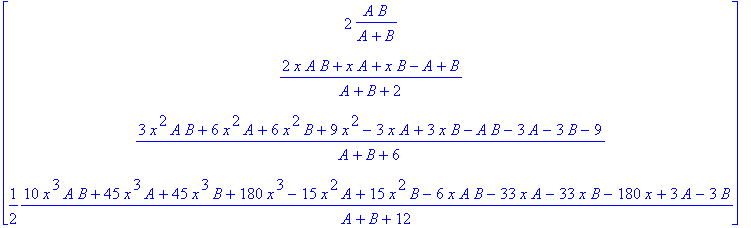We now plot the graphs of the first nine Krall polynomials. Observe that these polynomials are not symmetric, if A is not equal to B.

> with(plots);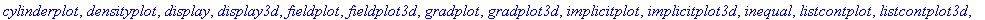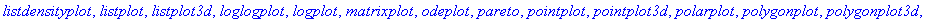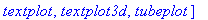> kp0:= plot(K(0,1,10,x), x=-1..1):
kp1:= plot(K(1,1,10,x), x=-1..1):
kp2:= plot(K(2,1,10,x), x=-1..1):
kp3:= plot(K(3,1,10,x), x=-1..1):
kp4:= plot(K(4,1,10,x), x=-1..1):
kp5:= plot(K(5,1,10,x), x=-1..1):
kp6:= plot(K(6,1,10,x), x=-1..1):
kp7:= plot(K(7,1,10,x), x=-1..1):
kp8:= plot(K(8,1,10,x), x=-1..1):
display(array(1..3,1..3,
[[kp0,kp1,kp2],[kp3,kp4,kp5],[kp6,kp7,kp8]]));In the case A=B the Krall polynomials K(n,A,A, . ) coincide with the Legendre type polynomials P(n,A, . ) corresponding to the fourth-order Legendre type differential equation (cf. [V1] and section 5 of [V2] for details). These polynomials are symmetric: they are even or odd depending on the degree of the polynomial.

We list the first nine Legendre type polynomials.

> array(1..9,1..1,[[K(0,A,A,x)],[sort(simplify(K(1,A,A,x)))],
[sort(simplify(K(2,A,A,x)))],[sort(simplify(K(3,A,A,x)))],
[sort(simplify(K(4,A,A,x)))],[sort(simplify(K(5,A,A,x)))],
[sort(simplify(K(6,A,A,x)))],[sort(simplify(K(7,A,A,x)))],
[sort(simplify(K(8,A,A,x)))]]);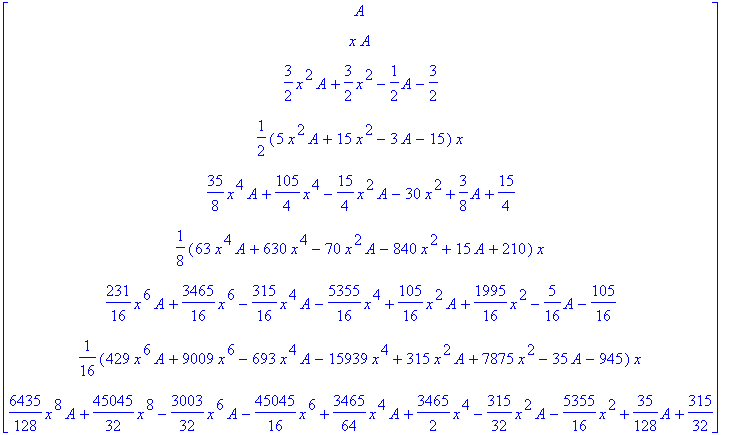We plot the first nine graphs in the case A=B=1.

> lp0:= plot(K(0,1,1,x), x=-1..1):
lp1:= plot(K(1,1,1,x), x=-1..1):
lp2:= plot(K(2,1,1,x), x=-1..1):
lp3:= plot(K(3,1,1,x), x=-1..1):
lp4:= plot(K(4,1,1,x), x=-1..1):
lp5:= plot(K(5,1,1,x), x=-1..1):
lp6:= plot(K(6,1,1,x), x=-1..1):
lp7:= plot(K(7,1,1,x), x=-1..1):
lp8:= plot(K(8,1,1,x), x=-1..1):
display(array(1..3,1..3,
[[lp0,lp1,lp2],[lp3,lp4,lp5],[lp6,lp7,lp8]]));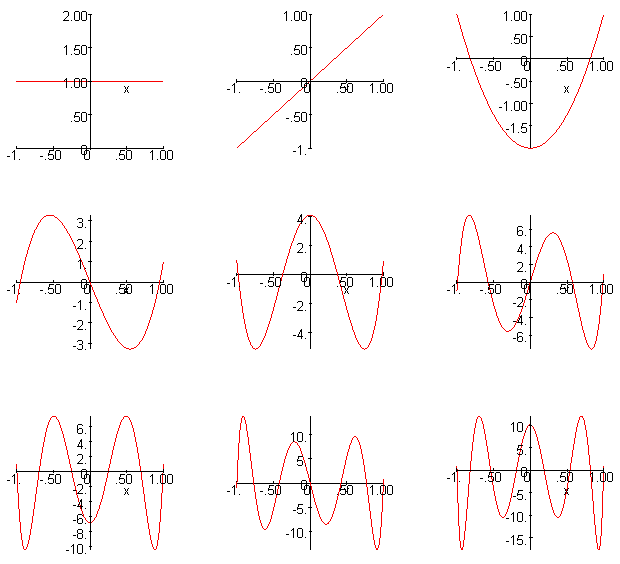4. The Weighted Sobolev Space

In the space W(-1,1) the following scalar product wip(. , .) is given, which is defined by means of a so called Dirichlet integral plus some boundary values.

Definition of the scalar product wip( . , . ) in W(-1,1):
(u,v - functions in W(-1,1), A,B - positive parameters)

We shall mention that the weight functions corresponding to the second and the third derivatives in the integral below vanish at the boundary of (-1,1). This corresponds with the fact that the sixth-order Legendre type differential equation is singular, since the weights in our scalar product are given by the coefficients of the differential operator.

Observe again that this scalar product wip( . , . , A,B) depends on the choice of the positive parameters A,B.

The following definition gives the real version of the scalar product. Since we are going to study only real-valued functions, it suffices to introduce this simpler version, in general we have to replace 'diff(v,x$k)' by 'conjugate(diff(v,x$k))' for k=0..3.

> wip := (u,v,A,B) -> int( u * v
+ (6*(A+B+2*A*B)*(1-x^2)+12*B*(1-x)+12*A*(1+x)+24)
* diff(u,x) * diff(v,x)
+ (1-x^2)*(12+(3*A+3*B+6)*(1-x^2))
* diff(u,x$2) * diff(v,x$2)
+ (1-x^2)^3 * diff(u,x$3) * diff(v,x$3), x=-1..1)
+ 1/A * subs( x=-1, u) * subs( x=-1, v)
+ 1/B * subs( x=1, u) * subs( x=1, v);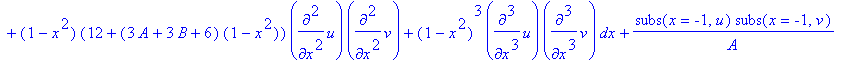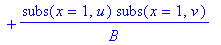In [V2], Theorem 2.1 we have obtained the following result, in which we use the notation ||f||_J := sup { |f(x)| , x in J }for continuous functions f: (-1,1) -> C and compact intervals J in (-1,1).

Theorem. The space (W(-1,1), wip( . , . )) is a Hilbert space and for every compact interval J in (-1,1) there exists a constant C(J) > 0 such that

||u||_J + ||u'||_J + ||u''||_J <= C(J) wip(u,u)^(1/2)

holds for all u in W(-1,1).

This weighted Sobolev inequality extends the well known Sobolev inequality from the space W^2_3(-1,1). It is the essential tool to deduce locally uniform expansions for the functions in W(-1,1) and their first two derivatives from weighted Sobolev norm expansions.

Examples: We give some examples for evaluations of the scalar product.

> wip(x,(1-x)^2*ln(1-x),A,B);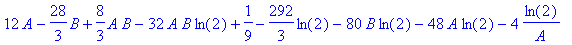Especially we can establish that the Krall polynomials are pairwise orthogonal with respect to the scalar product wip( . , . ).
This result is an easy consequence of the considerations in
[V2], since it is proved that the Krall polynomials are the eigenfunctions of an unbounded self-adjoint operator in the Hilbert space W(-1,1).

> wip(K(0,A,B,x),K(0,A,B,x),A,B);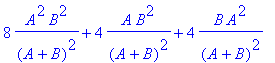> simplify(%);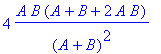> simplify(wip(K(1,A,B,x),K(2,A,B,x),A,B));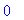> wip(K(3,1,10,x),K(7,1,10,x),1,10);We shall now consider some typical examples of functions in the weighted Sobolev space W(-1,1).

Examples:

(a) Clearly, in addition to every polynomial also every u in C^3[-1,1] is a function in the space W(-1,1). More generally, W^2_3(-1,1) is a subspace of W(-1,1).

(b) In general, every function u in W(-1,1) can continuously be extended onto the closed interval [-1,1] (see Lemma 3.1 of [V2]). However, the higher-order derivatives of u need not be bounded on the interval (-1,1). To illustrate this effect we introduce the following function g, which is in W(-1,1) and has an unbounded second derivative near x=1.

> g := x -> (1-x)^2*ln(1-x);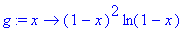> diff(g(x), x);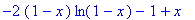> diff(g(x), x$2);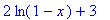We shall plot the graphs of g and its first three derivatives. > gp0:= plot( g(x), x=-1..1 ): gp1:= plot( diff(g(x),x), x=-1..1 ): gp2:= plot( diff(g(x),x$2), x=-1..1):
gp3:= plot( diff(g(x),x$3), x=-1..1): display(array(1..2,1..2, [[gp0,gp1],[gp2,gp3]]));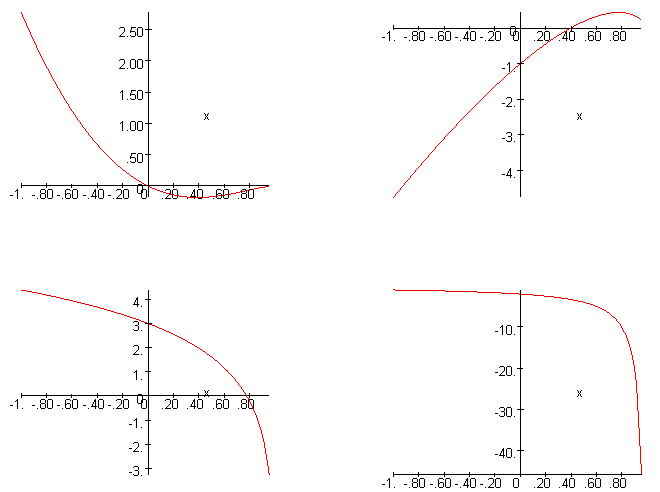(c) A second example will display the fact, that even the first derivative of a function in W(-1,1) may be unbounded. > h:= x-> int(ln(1-ln(1-t)),t=-1..x);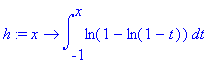> diff(h(x),x);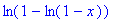> diff(h(x),x$2);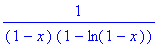> simplify(diff(h(x),x$3));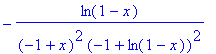> hp0:= plot( h(x), x=-1..1 ): hp1:= plot( diff(h(x),x), x=-1..1 ): hp2:= plot( diff(h(x),x$2), x=-1..1):
hp3:= plot( diff(h(x),x$3), x=-1..1 ): display(array(1..2,1..2, [[hp0,hp1],[hp2,hp3]]));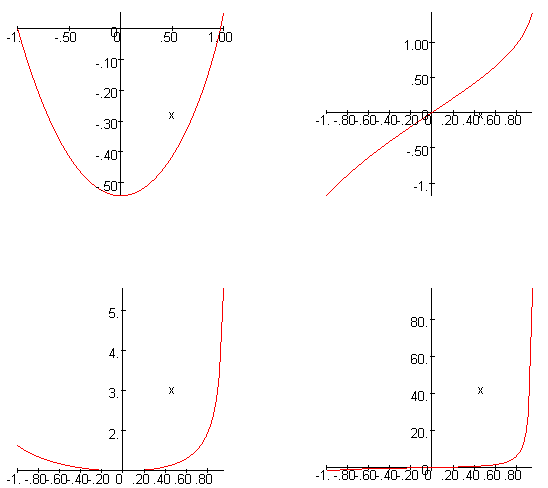-((1-x^2)^3 u''')''' + ((1-x^2)(12+(3A+3B+6)(1-x^2)) u'')'' - (\Pi(x)u')' + u = \lambda u, 24 B u''(1) + 24 (A+1) B u'(1) + u(1) = \lambda u(1), 24 A u''(-1) - 24 A (B+1) u'(-1) + u(-1) = \lambda u(-1), in which \Pi(x) = 6 (A+B+2AB) (1-x^2) + 12 B (1-x) + 12 A (1+x) + 24, \lambda denotes the eigenvalue parameter, and u: (-1,1) -> C is a function in W(-1,1), such that the derivatives u^(i) are locally absolutely continuous in the interval (-1,1) for every i = 0,...,5 (for details cf. [V2], Theorems 4.2 and 6.1). The eigenvalue \lambda(n,A,B) corresponding to the nth Krall polynomial K(n,A,B, . ) is given by \lambda(n,A,B) = n(n-1)(n-2)(n-3)(n-4)(n-5) + 18 n(n-1)(n-2)(n-3)(n-4) + 3 (A+B+32) n(n-1)(n-2)(n-3) + 24 (A+B+7) n(n-1)(n-2) + 3 (4AB+14A+14B+24) n(n-1) + 12 (2AB+A+B) n + 1. The above differential operator l(u) := -((1-x^2)^3 u''')''' + ((1-x^2)(12+(3A+3B+6)(1-x^2)) u'')'' - (\Pi(x)u')' + u is called the sixth-order Legendre type differential operator , it has been introduced by Littlejohn in [L1]. In [V2] we have shown, that the operator S: D(S) -> W(-1,1), Su := l(u), in which the domain of definition D(S) is a restriction of the maximal domain in W(-1,1) by means of the above boundary conditions, is an unbounded self-adjoint operator such that our boundary eigenvalue problem can equivalently be written in the form Su = \lambda u for u in D(S). This operator S has a purely discrete spectrum consisting of the simple eigenvalues \lambda(n,A,B) for n in N . So, the Krall polynomials form a complete orthogonal system of eigenfunctions in the Hilbert space W(-1,1) and every function u in W(-1,1) has a Fourier series expansion in terms of the normalized Krall polynomials (see [V2], Theorems 6.1 and 6.2). In general, norm expansions do not imply pointwise expansions. However, in Theorem 2.1 of [V2] we have proved a weighted Sobolev inequality for the functions in W(-1,1). An application of this inequality yields that the Fourier series and its first two derivatives converge locally uniformly on (-1,1) to the corresponding derivatives of the function u. The convergence of the Fourier series itself actually is uniform on the entire interval (see Theorem 6.4 of [V2]). In the sequel we introduce the Fourier series corresponding to the Krall polynomials in W(-1,1) and display its approximation property. Although the denseness of the linear span of the Krall polynomials in the weighted Sobolev space W(-1,1) can be proved independently of the spectral theory (cf. Lemma 5.3 and the references given in section 1 of [V2]), we shall point out, that the Fourier series provides a constructive approximation of the functions in W(-1,1). It is this constructiveness which enables our visualizations. Definition of the N-th partial sum of the Fourier series correponding to the Krall polynomials in (W(-1,1), wip( . , . ): (f - function in W(-1,1), N - degree of the partial sum, A,B - positive parameters, x - independent variable) As it was the case with the Krall polynomials and the scalar product in W(-1,1) this series depends on the choice of the parameters A,B > 0. For special applications below we will choose concrete values, e.g. A=1, B=10. > krallseries := (f,N,A,B,x) -> sum( '1/(wip(K(n,A,B,x),K(n,A,B,x),A,B))' * 'wip(f,K(n,A,B,x),A,B)' * 'K(n,A,B,x)', n=0..N);We calculate the first partial sums of the Fourier series of the function g, g(x) = (1-x)^2 ln(1-x), which has been defined in section 4: > seq(sort(simplify(krallseries(g(x),i,1,10,x))), i=0..3);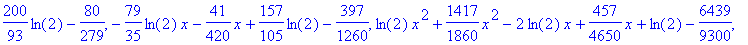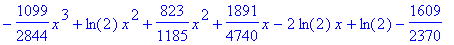At first, we visualize the function g and the first four partial sums of the approximating Fourier series corresponding to the Krall polynomials. > plot( [g(x), seq(krallseries(g(x),i,1,10,x),i=0..3)], x=-1..1, color=[black,blue,green,red,yellow], title=g(x) = (1-x)^2 ln(1-x) and the first partial sums of the Fourier series of g );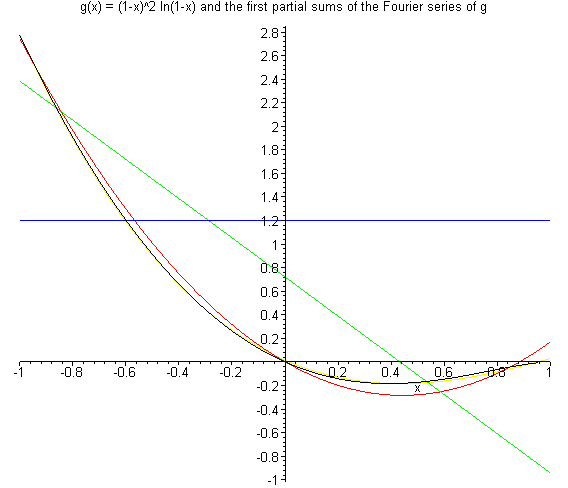Next we display the derivative g' and the derivatives of the first five partial sums of the approximating Fourier series with respect to the Krall polynomials. > diff(g(x),x);> plot( [diff(g(x),x), seq(diff(krallseries(g(x),i,1,10,x),x),i=0..4)], x=-1..1, color=[black,blue,green,red,yellow,pink], title=g' and the derivatives of the first partial sums of the Fourier series of g );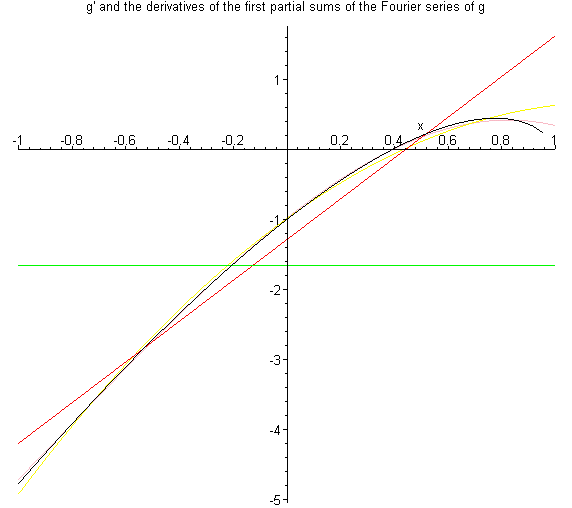We now visualize the second derivative g'' and the second derivatives of the first six partial sums of the approximating Fourier series corresponding to the Krall polynomials. We shall point out, that g'' is unbounded near the boundary x=1. > diff(g(x),x$2);> plot( [diff(g(x),x$2), seq(diff(krallseries(g(x),i,1,10,x),x$2),i=0..5)], x=-1..1,
color=[black,blue,green,red,yellow,pink,navy],
title=g'' and the second derivatives of the first partial sums of the Fourier series of g );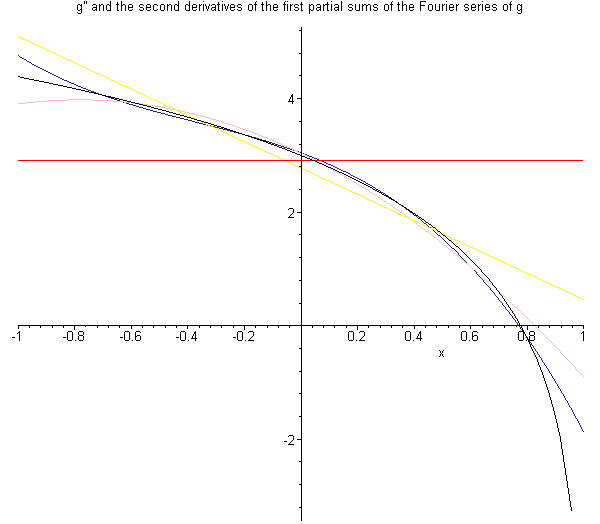Finally, we plot the third derivative of g and the third derivatives of the first seven partial sums of the Fourier series corresponding to the Krall polynomials.

> diff(g(x),x$3);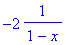> plot( [diff(g(x),x$3), seq(diff(krallseries(g(x),i,1,10,x),x$3),i=0..6)], x=-1..1, color=[black,blue,green,red,yellow,pink,navy,turquoise], title=g''' and the third derivatives of the first partial sums of the Fourier series of g );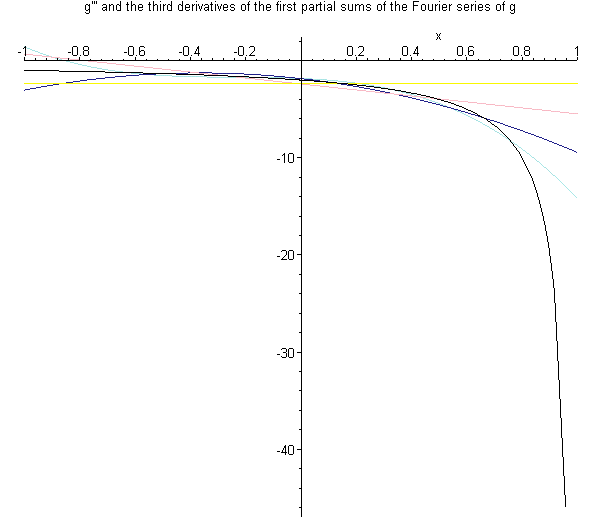Summary of the preceding four plots: > ep0:= plot( [g(x), seq(krallseries(g(x),i,1,10,x),i=0..3)], x=-1..1, color=[black,blue,green,red,yellow], title=g, g(x) = (1-x)^2 ln(1-x)  ): ep1:= plot( [diff(g(x),x), seq(diff(krallseries(g(x),i,1,10,x),x),i=0..4)], x=-1..1, color=[black,blue,green,red,yellow,pink], title=g' ): ep2:= plot( [diff(g(x),x$2), seq(diff(krallseries(g(x),i,1,10,x),x$2),i=0..5)], x=-1..1, color=[black,blue,green,red,yellow,pink,navy], title=g'' ): ep3:= plot( [diff(g(x),x$3), seq(diff(krallseries(g(x),i,1,10,x),x$3),i=0..6)], x=-1..1, color=[black,blue,green,red,yellow,pink,navy,turquoise], title=g''' ): display(array(1..2,1..2, [[ep0,ep1],[ep2,ep3]]));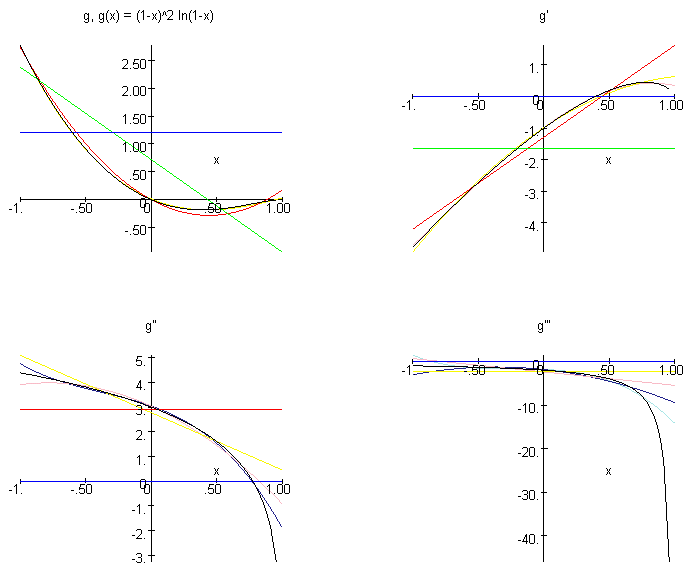The first three plots in this summary visualize the result on the locally uniform expansion of the functions in W(-1,1) in terms of their Fourier series, which is proved in Theorem 6.4 of [V2]: Theorem. Every function u in W(-1,1) and the first two derivatives u', u'' are locally uniformly approximated on the interval (-1,1) by the corresponding derivatives of the partial sums of the Fourier series corresponding to the Krall polynomials. As the remark to Theorem 6.4 shows, the approximation of u indeed holds uniformly on the entire interval (-1,1). The functions u in W(-1,1) admit their boundary values in 1 and -1 (see Lemma 3.1 of [V2]) and in this sense the expansion in terms of the Krall polynomials also holds in x=1 and x=-1. On the other hand the first and the second derivative of the functions in W(-1,1) may be unbounded near the boundary of (-1,1) and therefore in general these functions can not be approximated uniformly on (-1,1) by polynomials. For instance, our function g above, g(x) = (1-x)^2 ln(1-x), has an unbounded second-order derivative, while the function h from section 4 even has an unbounded first-order derivative. The fourth plot above displays g''' and the third derivatives of the partial sums of the Fourier series. In this case the approximation seems to be worse and indeed, the theory in [V2] does not give such a result for abitrary functions in the weighted Sobolev space W(-1,1). If, additionally the function u is in the domain of definition D(S) of the corresponding self-adjoint operator S, then our Theorem 6.5 in [V2] gives the following result on the locally uniform simultaneous approximation of the first eight derivatives: Theorem. Every function u in D(S) and its first eight derivatives can locally uniformly be expanded in terms of the corresponding derivatives of the Fourier series corresponding to the Krall polynomials. Observe that the function g given by g(x) = (1-x)^2 ln(1-x) does not belong to the domain D(S). In a second visualization of the approximation property of the Krall polynomials we study the following function g2. > g2 := x -> (1-x^2)^2*ln(1-x^2);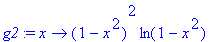The oscillation of the graph of g2 makes it necessary to consider partial sums up to the order 6 to obtain a suitable approximation. > plot( [g2(x), seq(krallseries(g2(x),i,1,10,x),i=0..7)], x=-1..1, color=[black,blue,green,red,yellow,pink,navy,turquoise,red], title=g2(x) = (1-x^2)^2 ln(1-x^2) and the first partial sums of the Fourier series of g2 );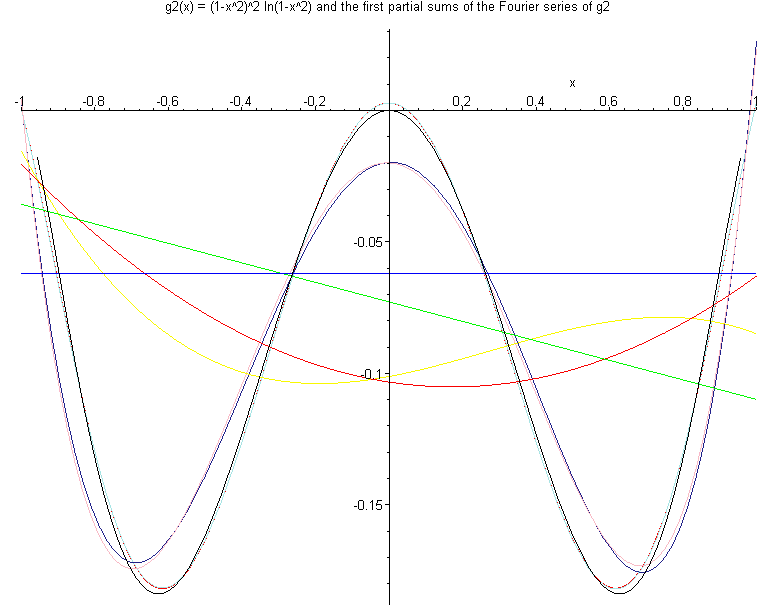The plot shows that the 4th partial sum (pink) nearly coincides with the 5th partial sum (navy), and that the 6th partial sum (turquoise) almost agrees with the 7th partial sum (red). This correponds with the fact, that the (normalized) odd order Fourier coefficients of g2 are small in relation to the even order Fourier coefficients: > seq( evalf(wip(g2(x),K(i,1,10,x),1,10)/wip(K(i,1,10,x),K(i,1,10,x),1,10),2), i=0..12);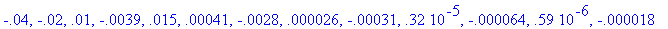The cause of this effect clearly is the symmetry of the function g2. Since g2 is an even function, since the Krall polynomials to the parameters A=1 and B=10 are almost even or odd , respectively, in dependence of their degree, and since the weighted Sobolev scalar product as well is nearly symmetric , we can expect such a behaviour. In the case A=B of the Legendre type polynomials we obtain full symmetry and therefore we exactly have wip(g2(x),K(i,1,1,x),1,1) = 0 for all odd i: > seq( evalf(wip(g2(x),K(i,1,1,x),1,1)/wip(K(i,1,1,x),K(i,1,1,x),1,1),2), i=0..12);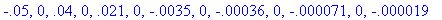We now turn back to the general Fourier series corresponding to the polynomials K(n,1,10, . ). Again, we obtain approximations of the derivative g2' as well by means of the derivatives of the Fourier series. > diff(g2(x),x);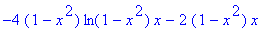> plot( [diff(g2(x),x), seq(diff(krallseries(g2(x),i,1,10,x),x),i=0..6)], x=-1..1, color=[black,blue,green,red,yellow,pink,navy,turquoise], title=g2' and the derivatives of the first partial sums of the Fourier series of g2 );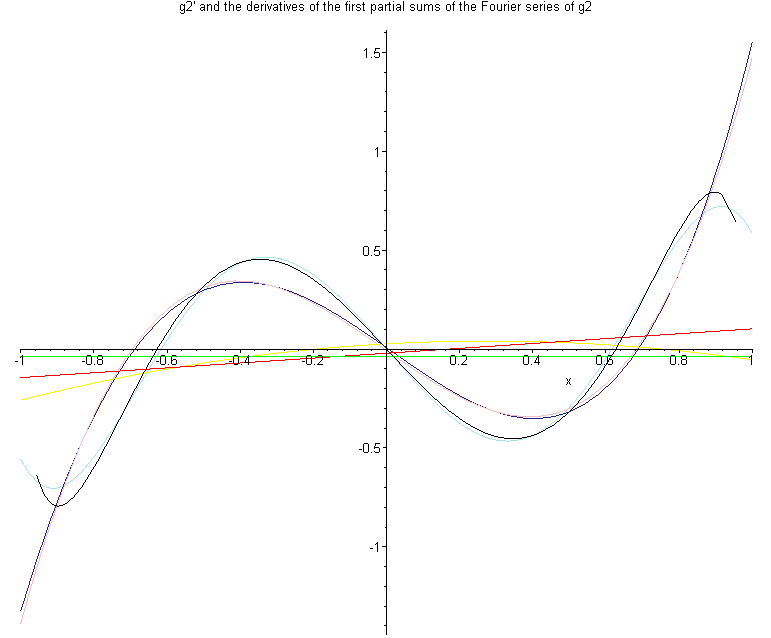Finally, we visualize the approximation of the second derivative g2''. > diff(g2(x),x$2);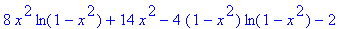> plot( [diff(g2(x),x$2), seq(diff(krallseries(g2(x),i,1,10,x),x$2),i=0..8)], x=-1..1,
color=[black,blue,green,red,yellow,pink,navy,turquoise,red,green],
title=g2'' and the second derivatives of the first partial sums of the Fourier series of g2 );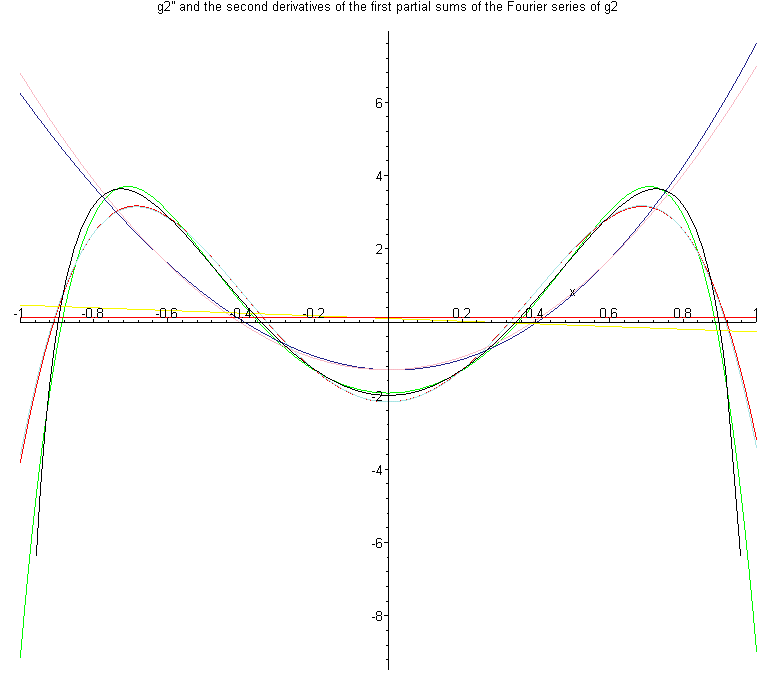Observe that the second derivative g2'' is unbounded at both end points of the interval (-1,1). But nevertheless, the second derivative of the eighth partial sum of the Fourier series corresponding to the Krall polynomials supplies a suitable locally uniform approximation of this function.

6. Conclusion

Simply speaking, mathematics is a matter of ideas.

The idea of orthogonal polynomials is quite old. It was in the year 1782 when A.M. Legendre has discovered the now called Legendre polynomials in a work on the attraction exerted by solids of revolution ( [Le1]). A few years later he established the orthogonality of these polynomials and used the fact that they satisfy an ordinary differential equation of second order (cf. [Le2] , [Le3]). Almost 200 years later, Littlejohn has discovered the Krall polynomials, which are closely connected with Legendre's polynomials, and thereby settled another member into the now large family of orthogonal polynomials ( [L1]).

In the meantime, the spectral theory of singular differential operators had well been developed. The origins of this idea can be found in the work of H. Weyl from the early 20th century. Already Weyl has studied the second order differential equations with orthogonal polynomial solutions in this respect (see e.g. his example on the Gaussian differential equation in §2 of his paper [W], which in some sense comprises Legendre's differential equation). Later, Titchmarsh (1940) and Glasmann (about 1950) have studied the classical orthogonal polynomials, including Legendre's polynomials, in the framework of spectral theory of differential operators in more detail (cf. here also the introduction of the survey paper [EL]). Their monographs Eigenfunction Expansions Associated with Second-Order Differential Equations ( [T]) and Theorie der linearen Operatoren im Hilbert-Raum ( [AG]) are still the basic references in this subject.

Till now, the interplay of orthogonal polynomials and spectral theory provides a broad field for mathematical activity. We hope, our visualizations given above may help to expound some more of the underlying ideas.

7. Historical References

[AG] N.I. Achieser and I.M. Glasmann : Theorie der linearen Operatoren im Hilbert-Raum , 8., erw. Aufl., hg. von H. Baumgärtel, Thun, Frankfurt am Main 1981.